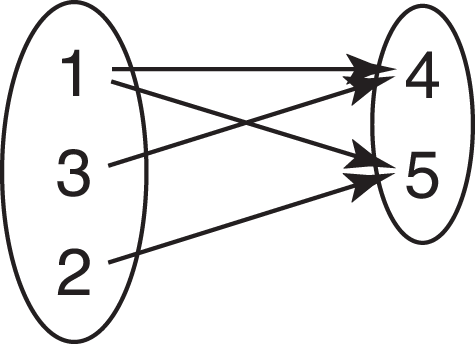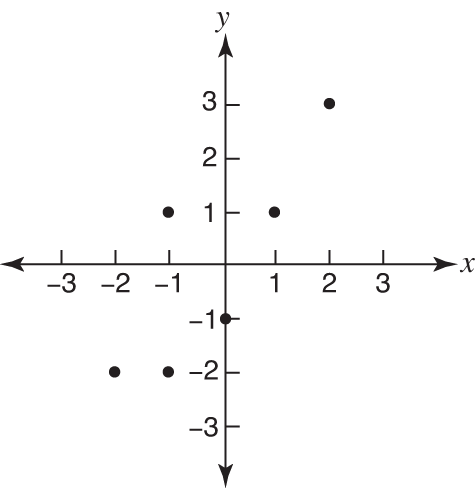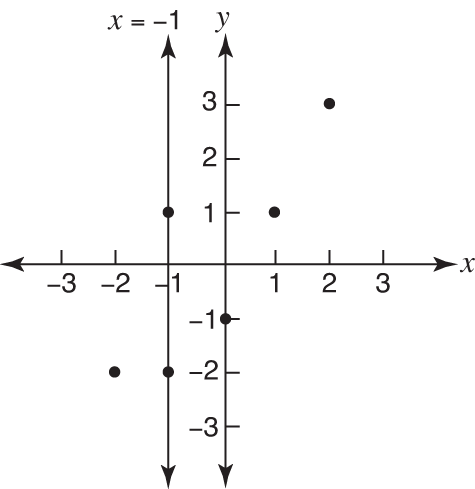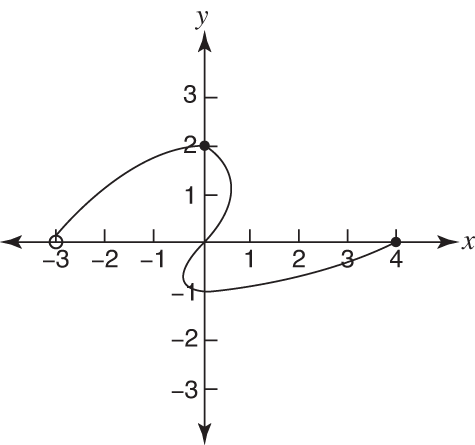## Basic Definitions

In algebra, there is frequently more than a single name for something. That's true of ordered pairs. The horizontal (or x) coordinates of a set of ordered pairs constitute the domain of a relation, while the range is determined by the vertical (or y) coordinates. A relation in which none of the domain numbers appears more than once is called a “function.” Following are definitions with which you should be familiar as you work with relations and functions.

#### Relation

A relation is a set of ordered pairs that can be represented by a diagram, graph, or sentence. Figure 1 shows several examples of relations.

Figure 1 Examples of relations.#### Domain and range

As noted, the set of all first numbers of the ordered pairs in a relation is called the domain of the relation. The set of all second numbers of the ordered pairs in a relation is called the range of the relation. The values in the domain and range are usually listed from least to greatest.

##### Example 1

Find the domain and range of item (a) in Figure 1.##### Example 2

Find the domain and range in item (b) of Figure 1.##### Example 3

Find the domain and range in item (c) of Figure 1.##### Example 4

Find the domain and range in item (d) of Figure 1.

The domain and range cannot be listed as in the preceding examples. In order to visualize the domain, imagine each point of the graph going vertically to the x‐axis. The points on the x‐axis become the domain (see Figure 2).

The domain now can be expressed as domain = { x|–3 < x ≤ 4}, which is read as “the set of x's such that x is greater than –3 and x is less than or equal to 4.”

To visualize the range, have all the points move horizontally to the y‐axis. The points on the y‐axis become the range (see Figure 3).

The range can be expressed as range = { y|–1 ≤ y ≤ 2}, which is read as “the set of y's such that y is greater than or equal to –1 and y is less than or equal to 2.”

Figure 2. The domain is horizontal.Figure 3. The range is vertical.##### Example 5

Find the domain and range in item (e) of Figure 1.

Since any value for x produces a y‐value and any value for y produces an x‐value,#### Function

A relation in which none of the domain values are repeated is called a function.

##### Example 6

Which of the examples given in Figure 1 are functions?

1. {(4,1), (3,2), (–1,6)}

This is a function, since domain values are not repeated.

2. The example, shown in Figure 4, is not a function.

The diagram represents the set of ordered pairs {(1,4), (1,5), (3,4), (2,5)} and (1,4) and (1,5) repeat domain values.

3. The example, shown in Figure 5, is not a function.

The graph represents the set of ordered pairs {(–2,–2), (–1,–2), (–1,1), (0,–1), (1,1), (2,3)}, and (–1,–2) and (–1,1) repeat domain values.

Notice that the vertical line x = –1 would pass through two points of the graph, as shown in Figure 6.

A vertical line that passes through (intersects) a graph in more than one point indicates ordered pairs that have repeated domain values and eliminates the relation from being called a function. This test for functions is called (not remarkably) the vertical line test.

4. The example shown in Figure 7 is also not a function. It fails the vertical line test.

5. y = 2 x + 3.

This is a function, since domain values are not repeated. When y = 2 x + 3 is graphed, it can be seen that the graph passes the vertical line test.

Figure 4. This is not a function.Figure 5. Like Figure 4, this is also not a function.Figure 6. Functions have no repeated x values.Figure 7. This fails the vertical line test and is, therefore, not a function.Back to Top
A18ACD436D5A3997E3DA2573E3FD792A